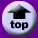Wilfrid Laurier University Official Web Site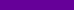Search :

 PC/CP200 Electronics Laboratory I Simple DC Circuits Objectives To understand the operation and applications of a voltage divider. To understand the operation and applications of a potentiometer or variable resistor. To understand the operation and applications of pull-up resistors. To understand the operation and applications of a current limiting resistor. Equipment digital multimeter, bench power breadboard, bare DIP switch or keypad various resistors, potentiometer, LEDs Procedure Resistors are frequently used to divide voltages into desired amounts to perform specific functions. A simple voltage divider is shown below to the left and a more complicated voltage divider is shown to the right. The numbered terminals are used as tap points to obtain the desired voltage(s). If nothing is connected to the terminals, the voltage divider is unloaded; if a resistor is connected to the terminals, the divider is loaded.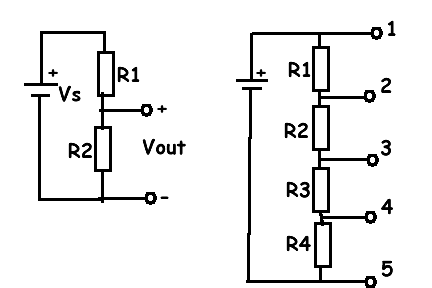Construct the simple voltage divider shown on the left, using two different-valued resistors with values of several kOhms each. Use +12V supply. Measure Vout, and verify that it satisfies the voltage divider formula Vout = Vs x R2/(R1+R2). Design and construct a voltage divider that divides an input voltage by three. Use +12V supply. Demonstrate and explain operation of this voltage divider to lab instructor. Construct the more complex voltage divider shown on the right under question 1. Use four different resistor values of several kOhms each (e.g. 3.3, 5.6, 2.2, 3.9), and a +12V supply. Measure the actual resistance for each of the resistors. Measure the voltage drop across each resistor. What percentage of the total voltage does each voltage drop represent? Measure the voltage from each tap point to ground. The tap points are the points numbered 1 through 5. How does this voltage divider work? Explain. A potentiometer or variable resistor is a voltage divider with a variable division ratio. The diagram on the left shows the symbols for a potentiometer. The diagram on the right (hopefully) makes clear the relationship between a potentiometer and voltage divider.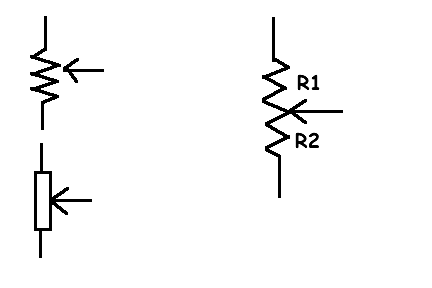The total resistance of the potentiometer, R1 + R2, is constant. The arrow represents a slider or knob which will vary the values of R1 and R2 subject to the constraint that the total resistance does not change. Use an ohmmeter to study the resistance of the (unconnected) potentiometer. Correlate your observations with the diagram above. Determine the minimum and maximum values of R1, R2, and R1+R2. Using the potentiometer instead of the resistors, construct the simple voltage divider shown in question 1. Observe the effect of the potentiometer setting on the value of Vout. What are the minimum and maximum attainable values of Vout? How do they compare to the supply voltage, Vs? Modify your circuit to add a "load" (e.g. 10 kOhms) to the center tap of the potentiometer. Repeat you measurements.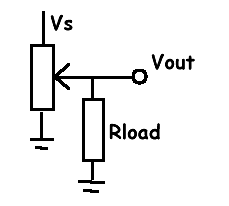Demonstrate and explain your results to the lab instructor. Potentiometers can be linear, i.e. R2 varies in proportion to the wiper's displacement. Potentiometers can also be logarithmic, i.e. logR2 varies in proportion to the wiper's displacement. The logarithmic potentiometers are popular in volume control circuits because the perception of loudness follows a logarithmic law. One of the applications of a resistor is to limit current. Consider the case of a circuit input which will be controlled by a switch. Proper implementation of switches is especially important in digital circuits. Consider the following circuits: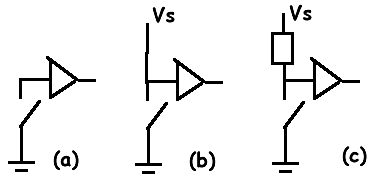In (a) the input to the buffer gate is directly connected to the switch. If the switch is closed, the input is connected to ground and the input is a stable low. However, when the switch is open, the input floats as it is not attached to any stable source. Although the input will tend to float high, any electrical noise could cause the input to go low. This is not a good circuit. In (b) the input to the buffer is directly connected to the supply voltage, Vs at +5V, to ensure that the input will not float when the switch is open. However, when the switch is closed, there will be a direct connection between power and ground or, in other words, a short circuit. The circuit will not work. There will be too much current, too much heat, goodbye wire! This is not a good circuit. In (c) the input to the buffer is connected to the supply voltage, Vs at +5V, through a resistor. This is called a pull-up resistor because it "pulls up" the input and ensures it does not float. When the switch is closed, the input to the buffer will be pulled low. Although current does flow from power to ground, it is severely limited by the resistor. In a typical digital circuit, Vs is +5V and the pull-up resistor is 10k or 47k Ohms. Construct a pull-up resistor circuit for a switch. The circuit does not have to feed any gate, simply measure the voltage Vout at the point the gate would be connected. When the switch is open, what is the voltage Vout? When the switch is closed, what is the voltage Vout? Calculate the current when the switch is closed. Points to ponder after the lab (include in post lab writeup) This circuit segment almost looks like a voltage divider. Is it a special case? There is also a pull-down resistor configuration. Can you figure out what it would look like? An LED (light emitting diode) is a semiconductor that emits photons when a current flows through it. An LED has very low internal resistance. If you do not use a current limiting resistor, too much current will flow through the LED and it will burn up. To a point, the higher the current, the brighter the LED. Most LED's have a current rating, which determines the size of the resistor you will need. The current rating tells you what the maximum allowable current for the part is. General purpose LED's handle currents in the 10-20mA range. LEDs are directional (have a positive and negative leg) and are forward biased because the current must flow from the positive to the negative. Directional components must be inserted in the correct orientation for the component to work. The symbol for the LED, a triangle with a bar at the negative end, may also include arrows going outward from the object to symbolize the light.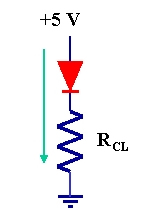(a)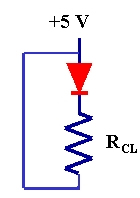(b)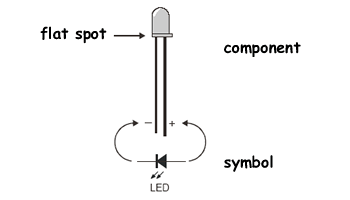The circuit would be set up as in figure (a). The circuit in figure (b) will not work because there is no current. (Do you understand why there is no current in this circuit? Explain.) Construct a test circuit to light up an LED. Assume the LED current rating is 10mA unless told otherwise by the lab supervisor. Show your calculations for determining the value of the current limiting resistor. Construct a simple circuit that has a switch and an LED that indicates the status of the switch. You decide if open|closed corresponds to on|off or off|on. Demonstrate and explain your circuit to the lab instructor. Use any remaining time you might have to practice for lab test 1. Before you leave the lab, have the lab instructor sign your lab notebook immediately after your last entry.

 Copyright © 2006 Terry Sturtevant, Wilfrid Laurier University Disclaimer